## 上下文无关文法 CFG

Context Free Grammar CFG 需满足：$\forall \alpha \rightarrow \beta \in P$，均有 $\alpha \in V$ 成立（即左侧不可以含有终止字符，但右侧可以含多个 symbols，而不是正则语言中的右侧只允许一个）。

ETFPid
ExpressionTermFactorPrimaryIdentifier

• 定义：生成树 / 派生树 parse tree：aka 分析树，语法树
• 对于字符串的另一种表达方式
•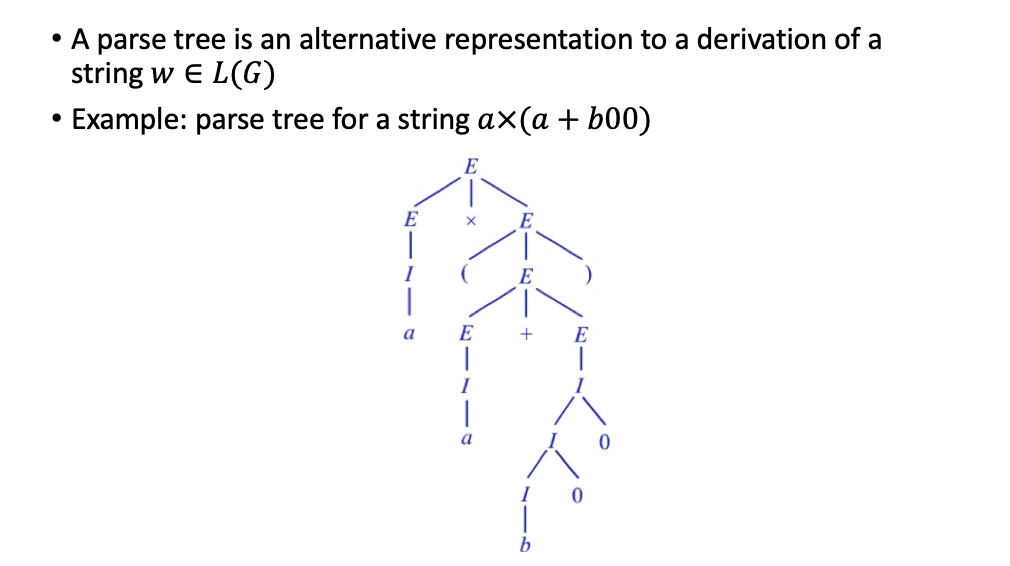### 派生方式与二义性

• 极左派生 Leftmost Derivations $\Rightarrow_{lm}$，在 $\alpha$ 派生过程中每一步都对最左变量进行替换（类似地有极右派生 Rightmost derivations），对应的规约有极左 / 右规约。

• 最右派生 aka 规范派生 Normal Derivation，规范派生产生的句型为规范句型 Normal Sentencial Form
• 二义性 Ambiguous Grammar：至少具两棵不同的派生树

• 判定 CFG 是否为 Ambiguous 是一个不可解问题

• 解决二义性的方法：增加优先级、增加标志等等

• 固有二义性 Inherent Ambiguity （并非所有语言都具备非二义性文法）

• 形如 $L = {0^n1^n2^m3^m}$ 的语言具备不同的派生树，具有固有的二义性，例如：
•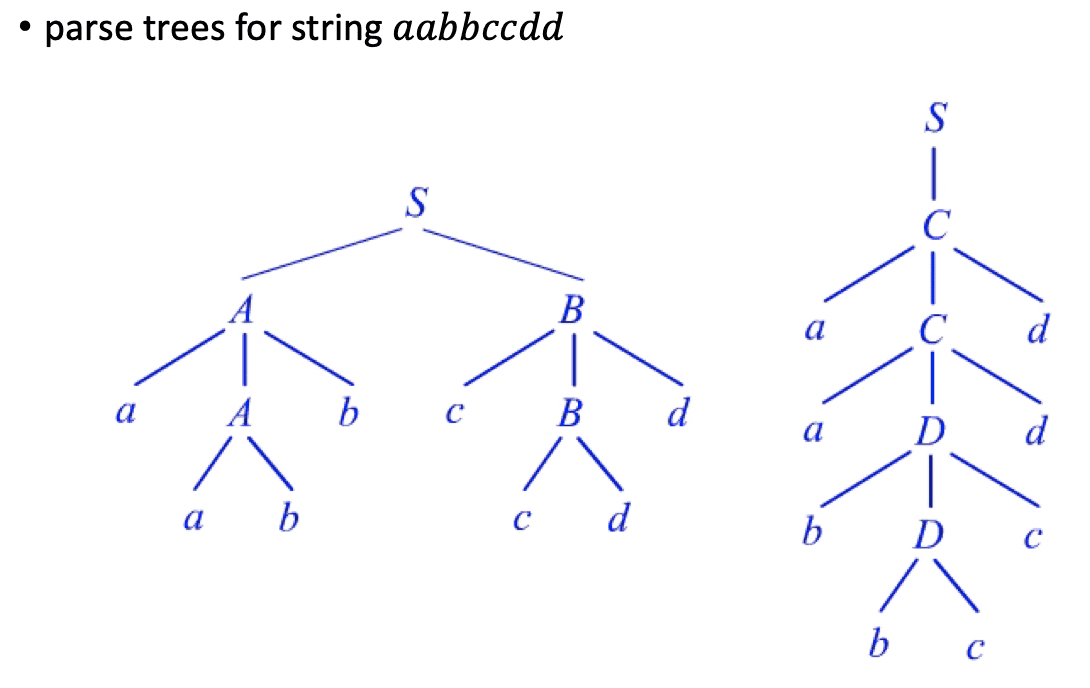### CFG 的化简

1. 消除无用符号：

有用符号：X 有效，当存在转移 $S \Rightarrow^* \alpha X\beta \Rightarrow^* w$

有用符号应当是可生成且可达的，非可达、非可生成的符号都需要被消去

• Generating: $X⇒^∗ w$ for some $w∈T^∗$
• Reachable: $S⇒^∗ αXβ$ for some ${α,β}⊆(V∪T)^∗$

Eg.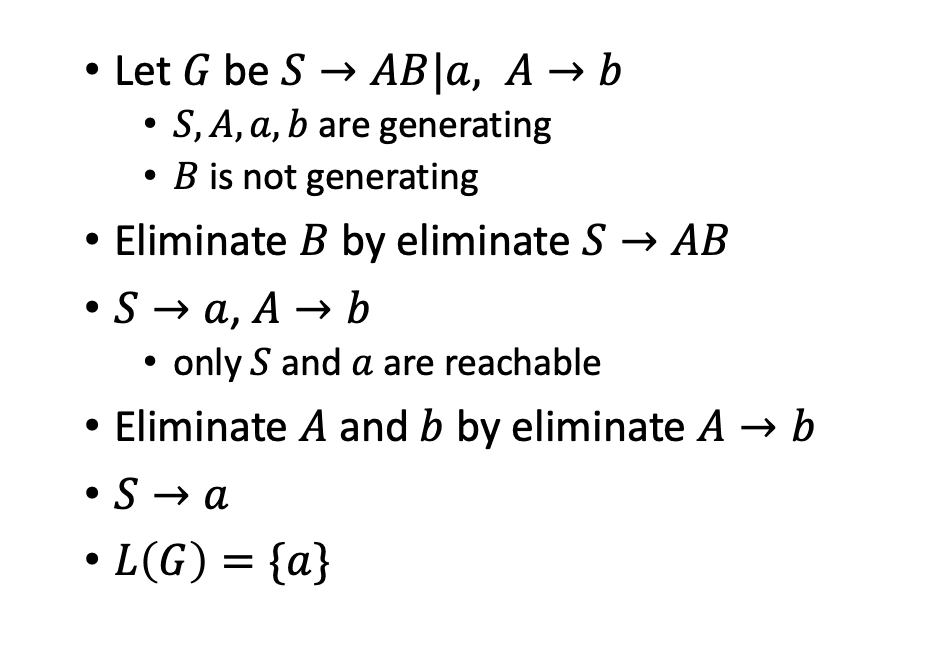2. 消除空转移

Nullable：A 是可空的当 $A \Rightarrow^* \varepsilon$

计算 nullables：先令 $n (G) = {A | A \rightarrow \varepsilon \in P}$，递归向上规约得到所有 $n (G)$

接下来做替换：若 A nullable，则 $A \rightarrow BAD$ 被替换成 $A \rightarrow BAD | BD$，最后消去所有 $A \rightarrow \varepsilon$

3. 消除单位转移 Unit Production (形如 $A \rightarrow B$)

先令 $n (G) = {(A,A)|A\in V}$，如果 $(A,B)\in G$ 且 $B\rightarrow C \in P$ 则将 (A, C) 添加至 G

接下来遍历 n (G)，每一对 (A, B) 如果：$B\rightarrow \alpha$ 不是一个单位转移，则添加 $A \rightarrow \alpha$ 添加至 $P_1$

### 范式

#### 乔姆斯基范式 Chomsky Normal Form CNF

$A \rightarrow BC$ ，$A \rightarrow a$，则为 CNF。

• CFL 转换为 CNF：处理所有推导结果长于 2 的，把 a 替换成新的 A，再增加 $A\rightarrow -a$
• 把所有长于 3 的级联分解成两个变量的转移，图示：
•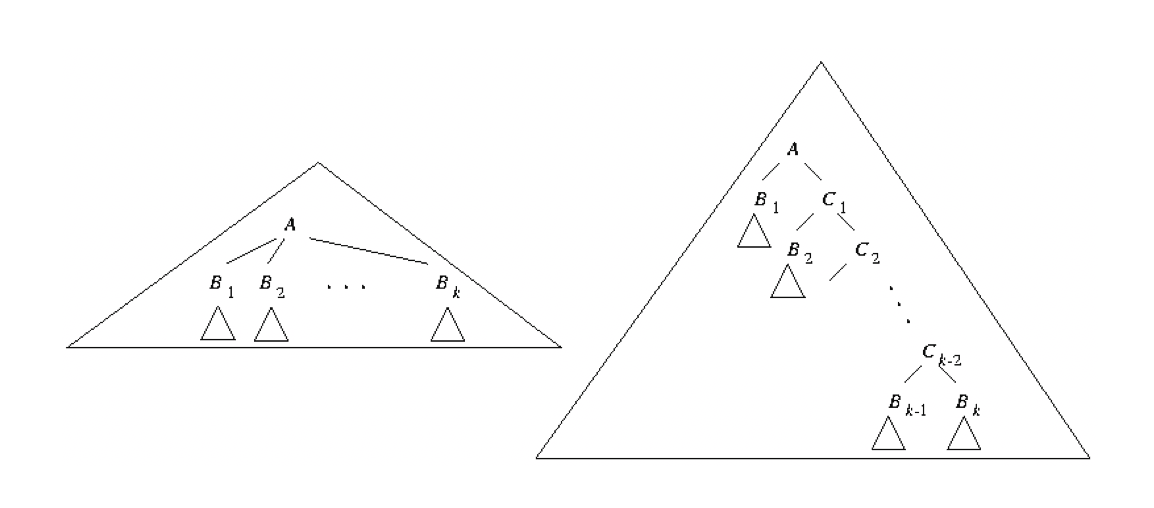### CFL 的泵引理

• 对于 CFL，存在一个 N 若 $|z| \gt n$ 则改写 $z = uvwxy$ 满足：
• $|vwx| \le N; |vx| > 0$
• 即有 $\forall i \ge 0, uv^iwx^iy \in L$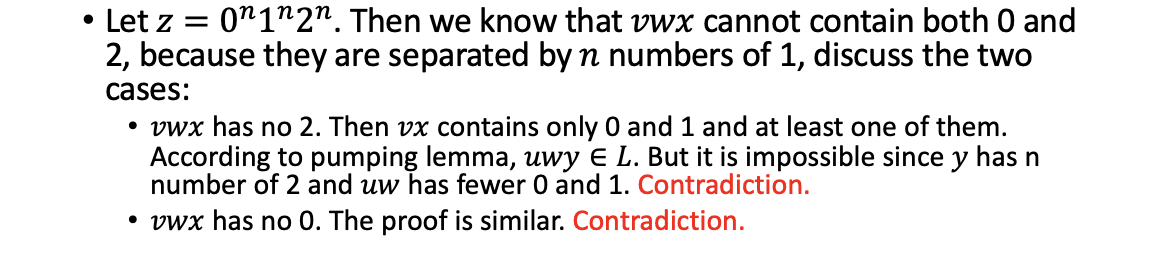## 下推自动机 Push Down Automaton PDA

PDA 与 CFG - 二型文法等价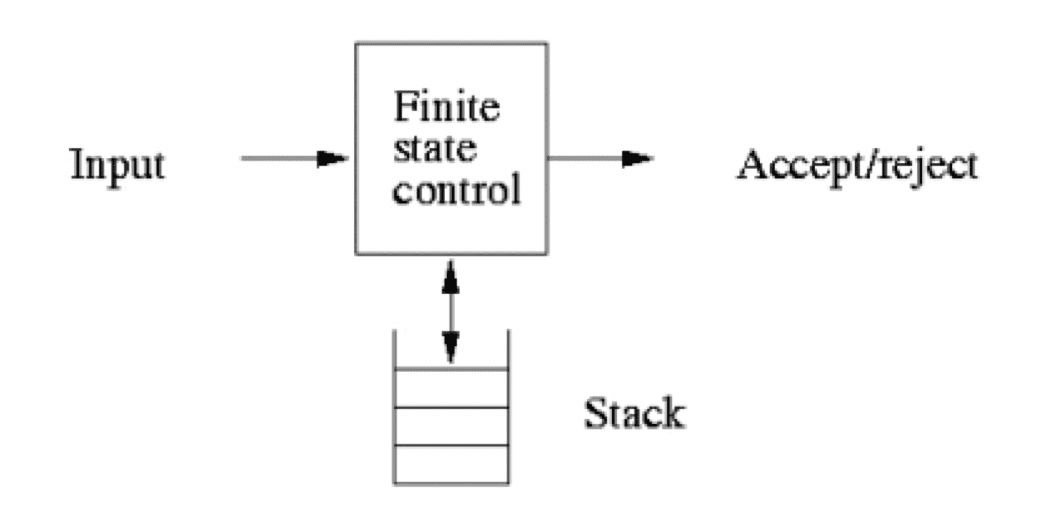• Q 状态集合；$\Sigma$ 输入字母表； $\Gamma$ 栈符号表；$Z_0$ 开始符号（栈底符号）$q_0$ 开始状态；$F$ 终止状态； $\delta$ 转移函数 ，形如：$\delta (q,a,Z) = {(p_1, \gamma_1), (p_2, \gamma_2)...}$ 为一次非空移动

• 形如：$\delta (q,\varepsilon,Z) = {(p_1, \gamma_1), (p_2, \gamma_2)...}$ 为一次空移动，此情况下无论输入内容是什么，都可以选择直接将状态变为 $p_n$。
• 一个例子：构造 $L_{wwr} = {ww^R | w\in {0,1}^*}$，其中 R 为倒序：

• PDA 在图形中表示如下，右侧表示栈 Z 在状态转移之前与之后的情况，栈顶位于左侧，栈底位于右侧：$Z_0$ 表示空栈
•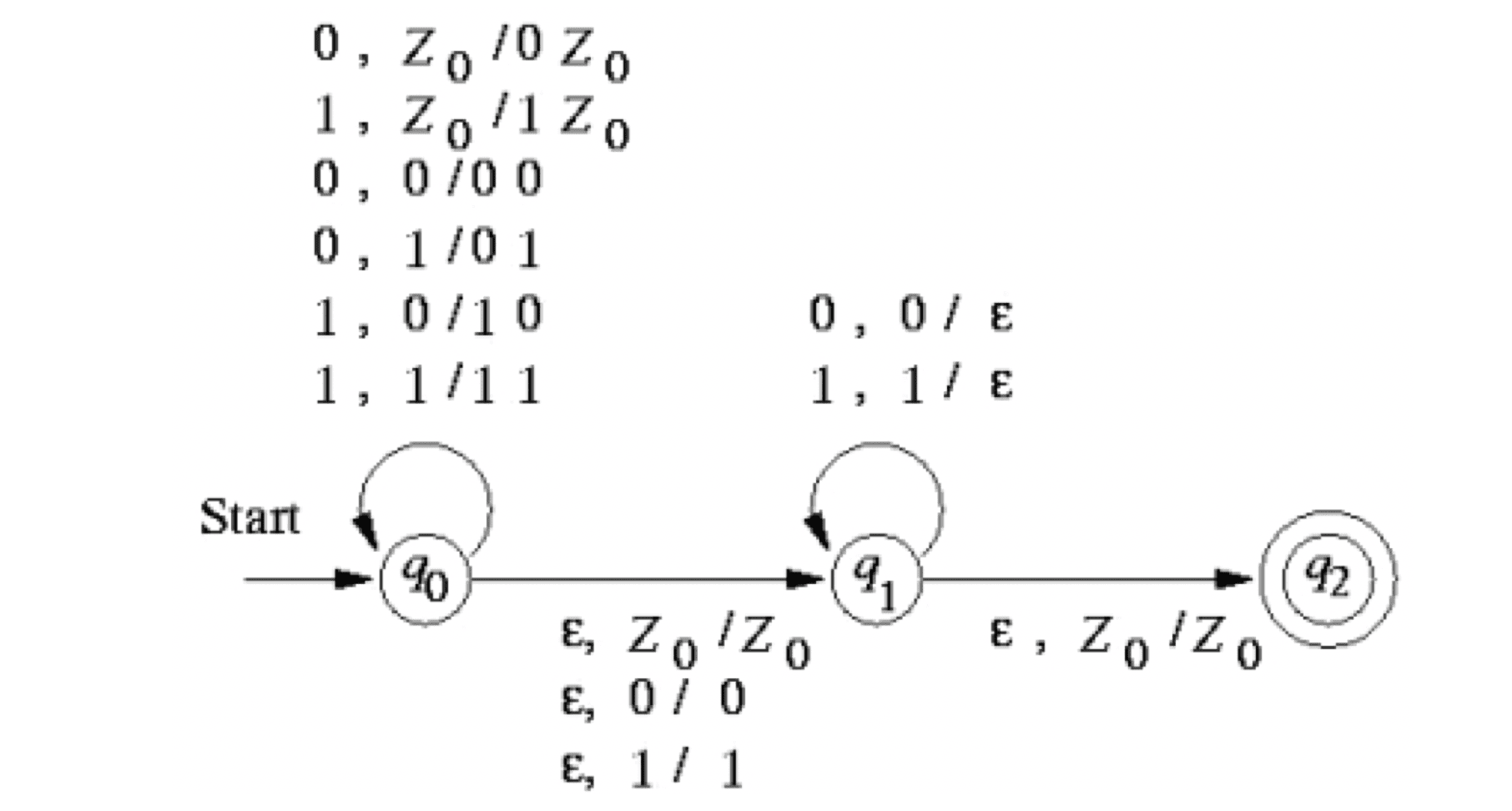• 确定下推自动机 DPDA：$\delta (q,a,X)$ 在 a 是 $\varepsilon$ 时总是空，且 $\delta (q,a,X)$ 非空则 $\delta (q,\varepsilon,X)$ 非空。

• Regular language $\subset$ L(DPDA) $\subset$ Context-Free language

### PDA 即时描述 Instantaneous Description

• 即时描述：$\forall (q,w,\gamma)\in (Q, \Sigma^*, \Gamma^*)$ 成为 M 的一个即时描述
• 一个非空移动的表示： $(q, aw, Z\beta) \vdash _M (p, w,\gamma\beta)$，其中 aw 为即将读入的字符串，M 之前位于状态 q，栈顶为 Z，进入状态 p 后 Z 被 $\gamma$ 替换。
• 同样有 $(\vdash_M)^*, (\vdash_M)^+,(\vdash_M)^n$ 的表达方式以表示特定次数的移动。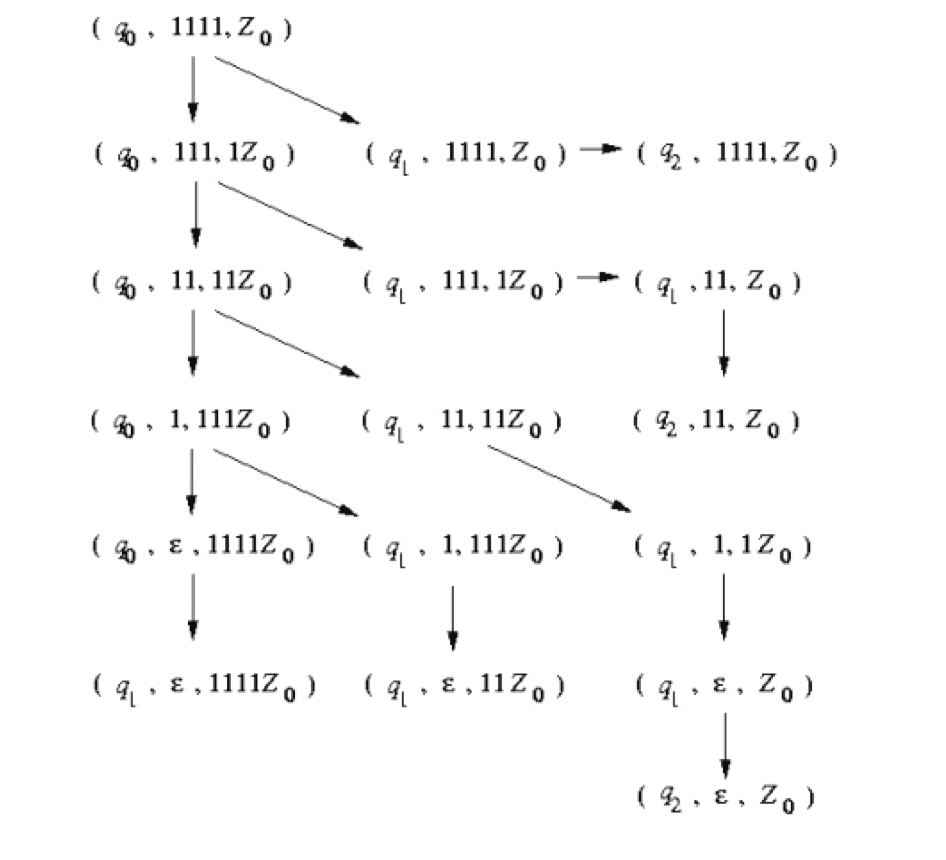###PDA 接受的语言

• 以终止状态接受：$L (P)={w ┤| (q_0,w,Z_0 ) ⊢^∗ (q,ϵ,α)∧q∈F}$
• 以空栈接受：$N (P)={w ┤| (q_0,w,Z_0 ) ⊢^∗ (q,ϵ,ϵ)}$

## CFG 与 PDA 的等价性

L 是 CFG 当且仅当能被一个 PDA 接受。（两种接收方式）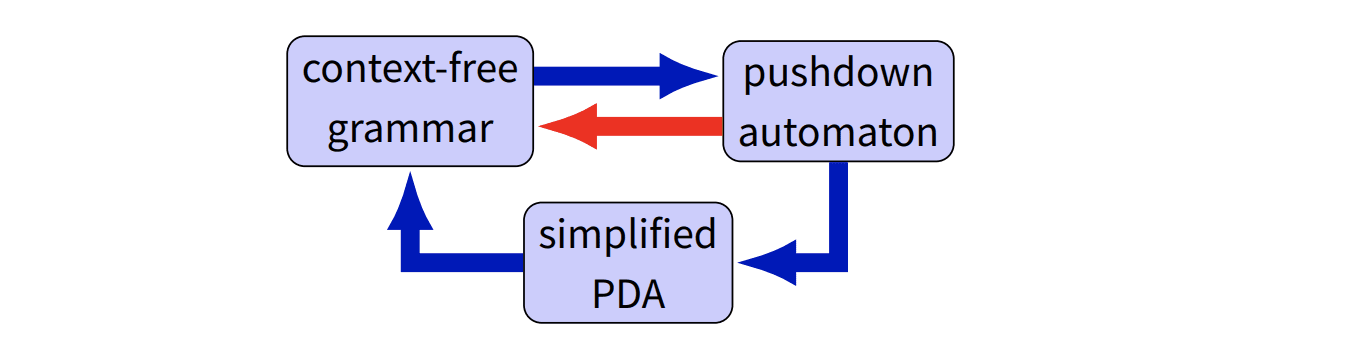#### CFG -> PDA

1. $xA\alpha\Rightarrow_{lm} x\beta\alpha$ 对应一个 PDA 的操作，在栈 Z 中压入非终止字符，在栈上有终止字符时便弹出

2. 此时可能会有非法的指令（在栈中一字符（包括 $\varepsilon$ 只能由一个字符替换）），可以进行指令分解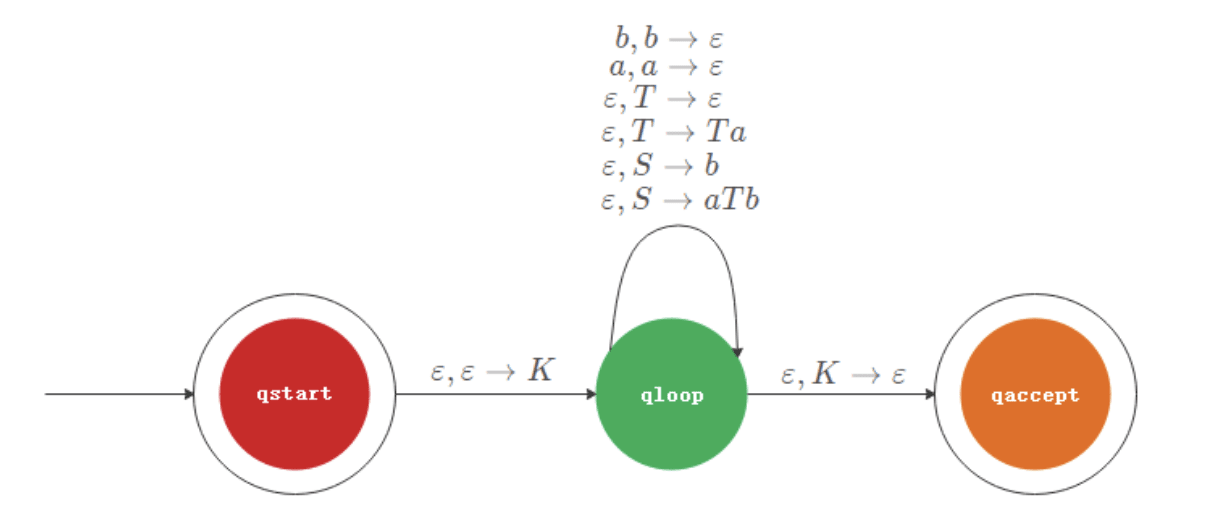分解 $\varepsilon, S \rightarrow aTb$ 之后自动机如图所示：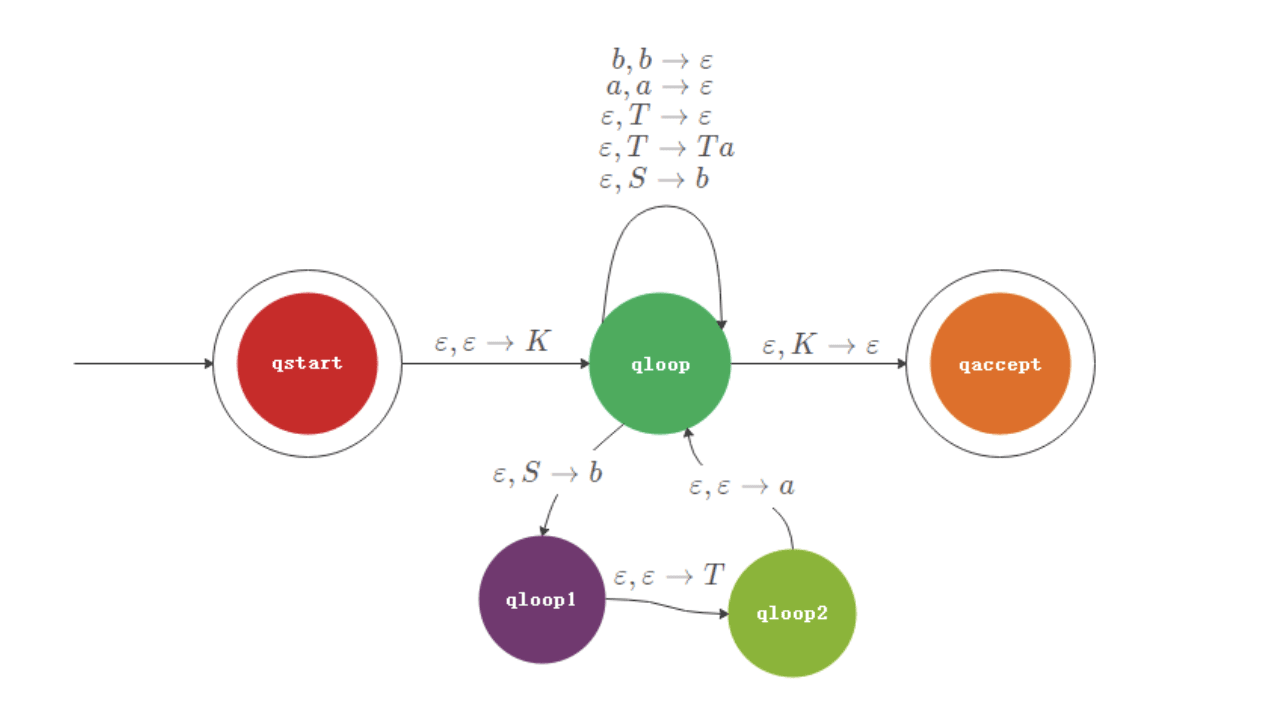以此类推进行指令分解。